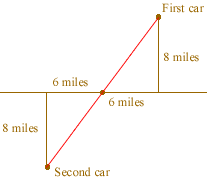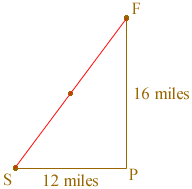Quandaries and Queries Two cars start off at the same point on a striaght highway facing opposite directions. Each car drives 6 miles, talkes a left turn, and drives for 8 miles. How far apart are the two cars. This is a question that has been going around work, it's been 10 years since I had geometry, and I can't remember how to figure this out. Can you help me, I want to be the one to tell everyone else what it is. Hi Nicole, I drew a diagram of the situation you described.then I redrew the diagram to form the right triangle FSP.Since this is a right triangle the theorem of Pythagoras says that |FS|2 = |SP|2 + |PF|2 Thus |FS|2 = 122 + 162 = 144 + 256 = 400 Hence |FS| is 20 miles. Cheers, Penny Go to Math Central